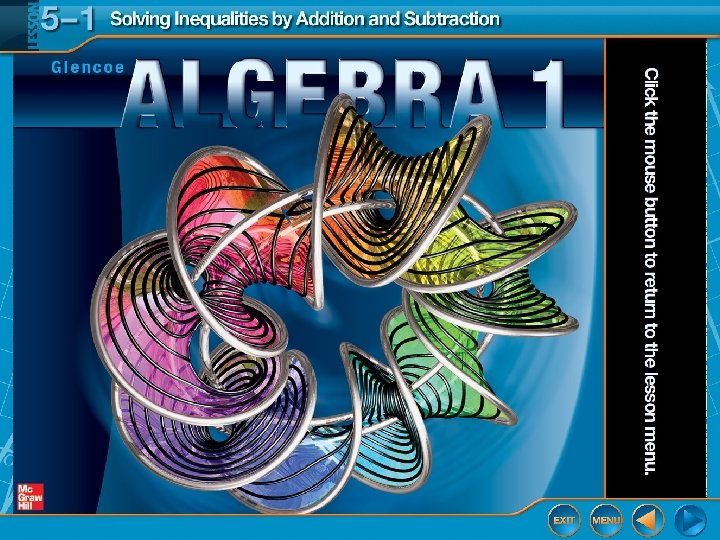Скачать презентацию Five-Minute Check over Chapter 4 CCSS Then Now New

f647a4282027675ebf36e1f66bb3f9f4.ppt

• Количество слайдов: 24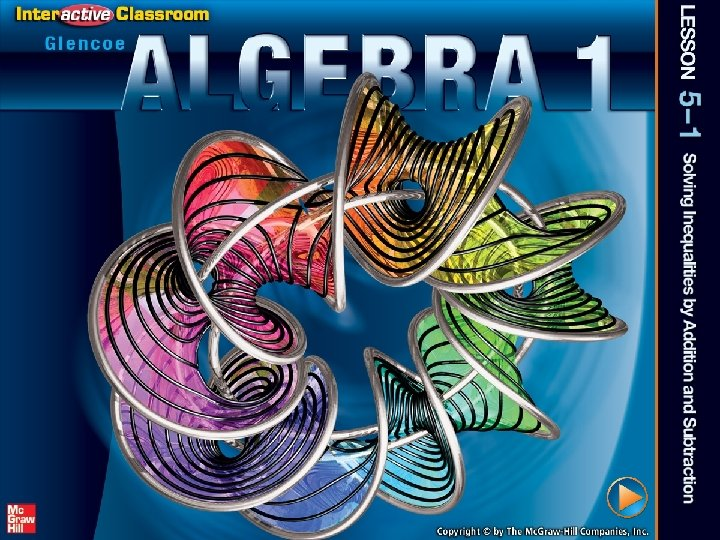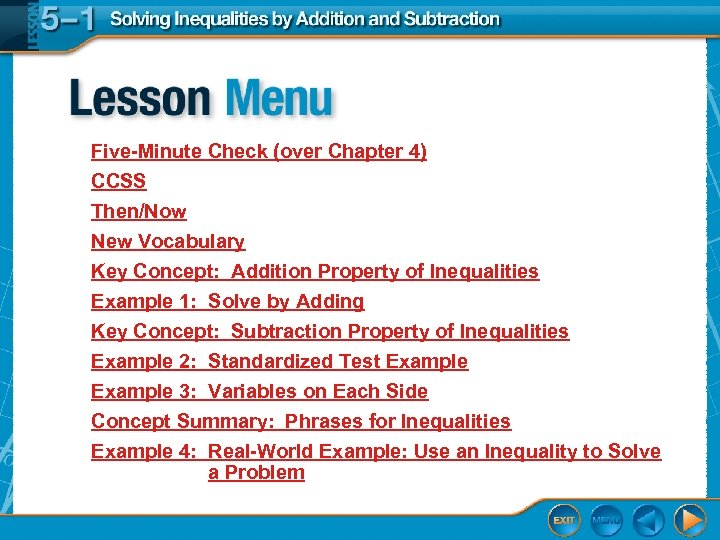Five-Minute Check (over Chapter 4) CCSS Then/Now New Vocabulary Key Concept: Addition Property of Inequalities Example 1: Solve by Adding Key Concept: Subtraction Property of Inequalities Example 2: Standardized Test Example 3: Variables on Each Side Concept Summary: Phrases for Inequalities Example 4: Real-World Example: Use an Inequality to Solve a Problem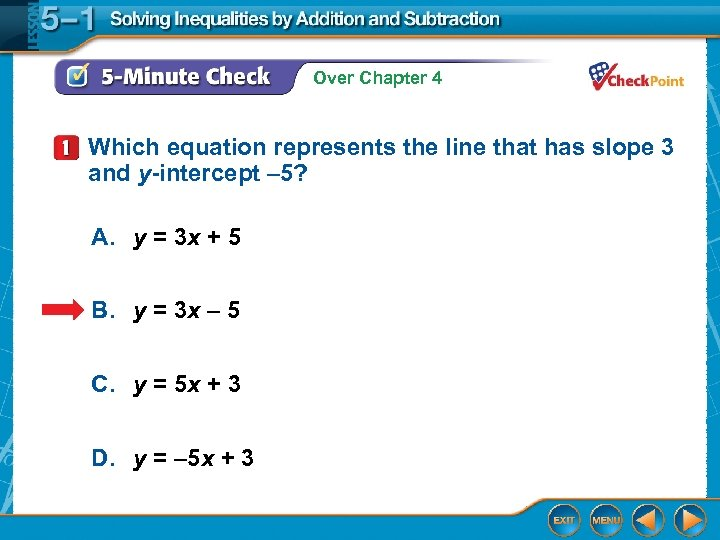Over Chapter 4 Which equation represents the line that has slope 3 and y-intercept – 5? A. y = 3 x + 5 B. y = 3 x – 5 C. y = 5 x + 3 D. y = – 5 x + 3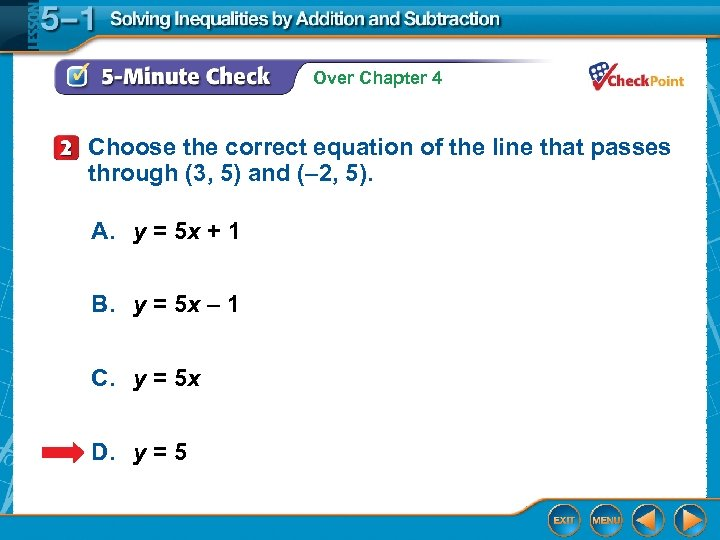Over Chapter 4 Choose the correct equation of the line that passes through (3, 5) and (– 2, 5). A. y = 5 x + 1 B. y = 5 x – 1 C. y = 5 x D. y = 5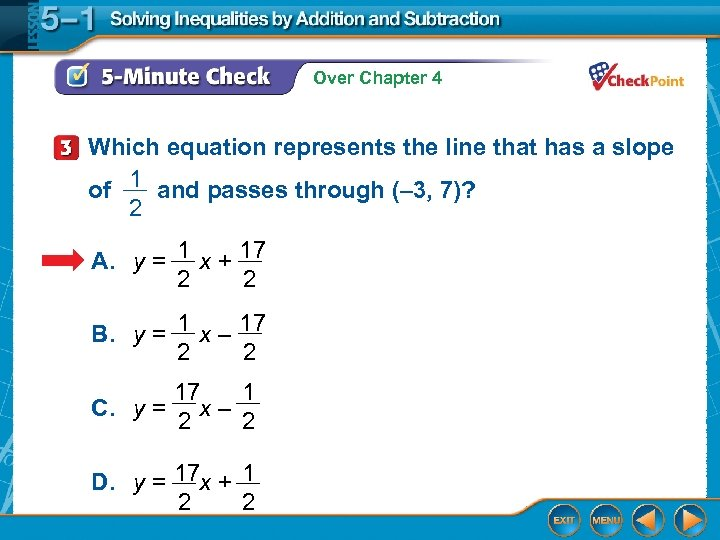Over Chapter 4 Which equation represents the line that has a slope 1 of __ and passes through (– 3, 7)? 2 1 17 A. y = __ x + __ 2 2 1 17 B. y = __ x – __ 2 2 C. y = __ 17 __ 1 2 2 x– 17 1 D. y = __ x + __ 2 2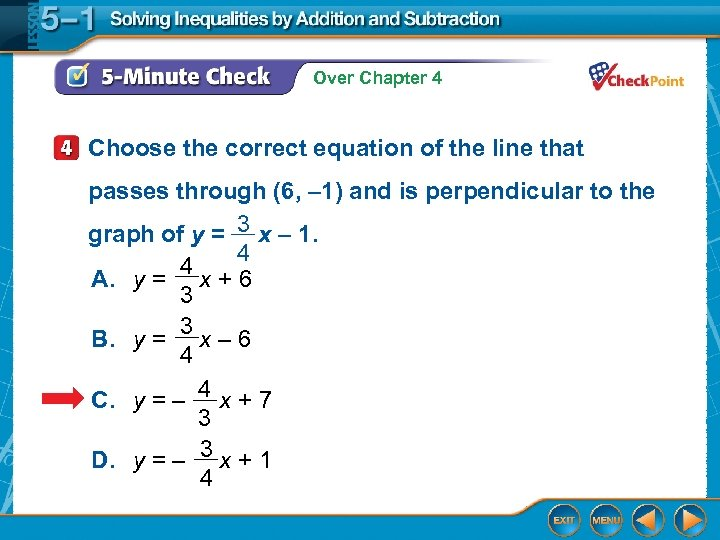Over Chapter 4 Choose the correct equation of the line that passes through (6, – 1) and is perpendicular to the 3 graph of y = __ x – 1. 4 __ 4 A. y = x + 6 3 __ 3 B. y = x – 6 4 4 C. y = – __ x + 7 3 3 D. y = – __ x + 1 4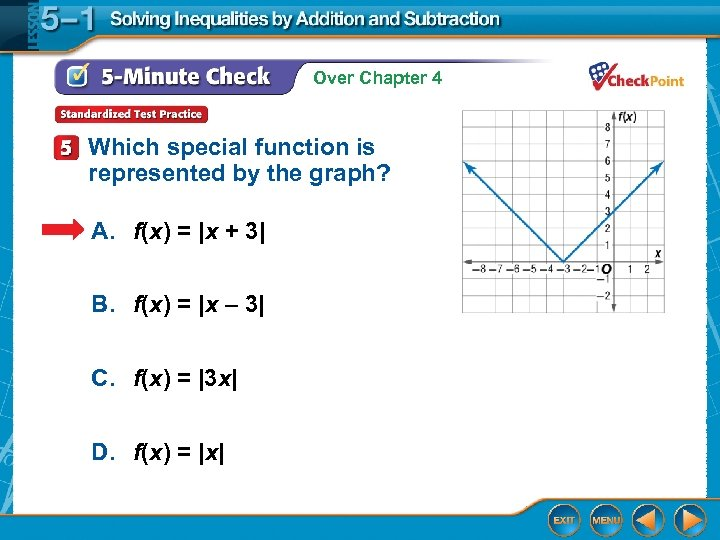Over Chapter 4 Which special function is represented by the graph? A. f(x) = |x + 3| B. f(x) = |x – 3| C. f(x) = |3 x| D. f(x) = |x|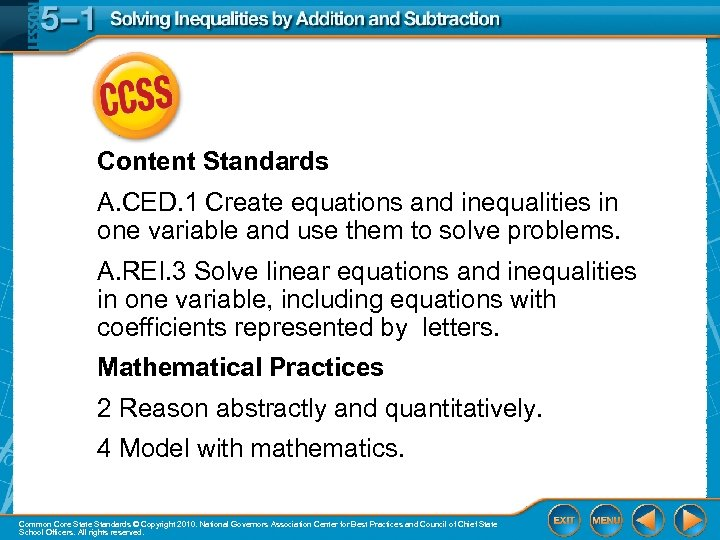Content Standards A. CED. 1 Create equations and inequalities in one variable and use them to solve problems. A. REI. 3 Solve linear equations and inequalities in one variable, including equations with coefficients represented by letters. Mathematical Practices 2 Reason abstractly and quantitatively. 4 Model with mathematics. Common Core State Standards © Copyright 2010. National Governors Association Center for Best Practices and Council of Chief State School Officers. All rights reserved.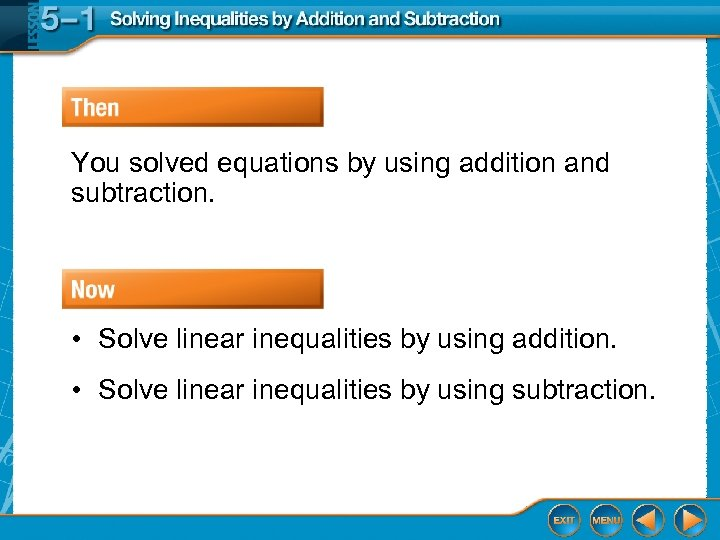You solved equations by using addition and subtraction. • Solve linear inequalities by using addition. • Solve linear inequalities by using subtraction.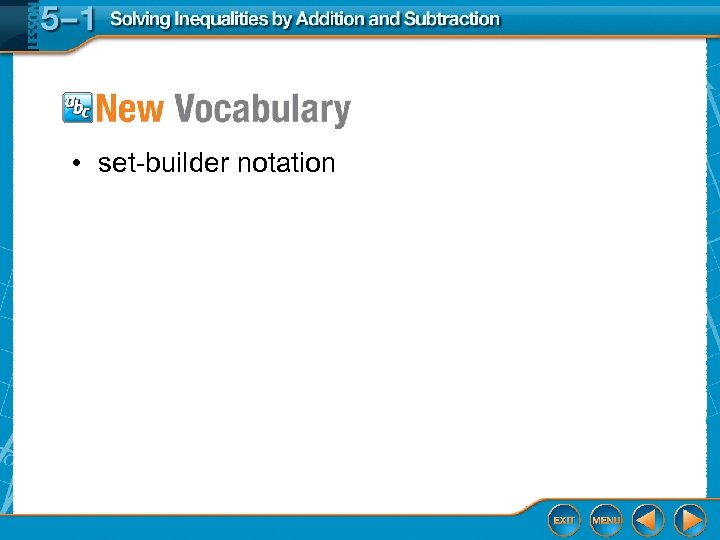• set-builder notation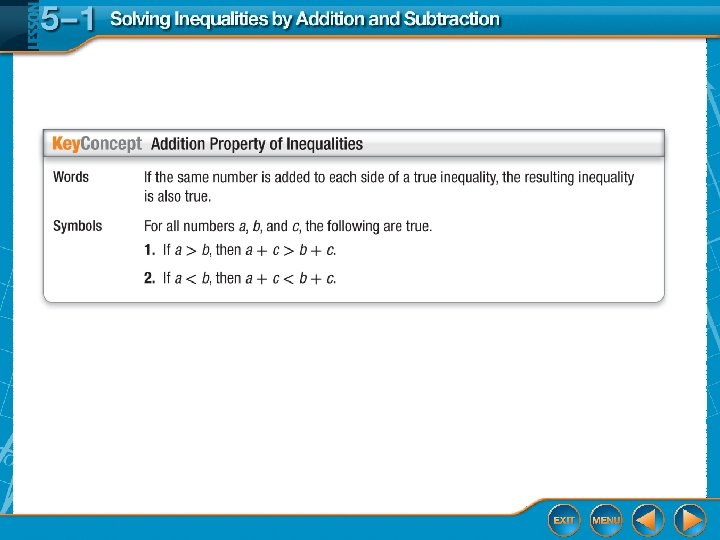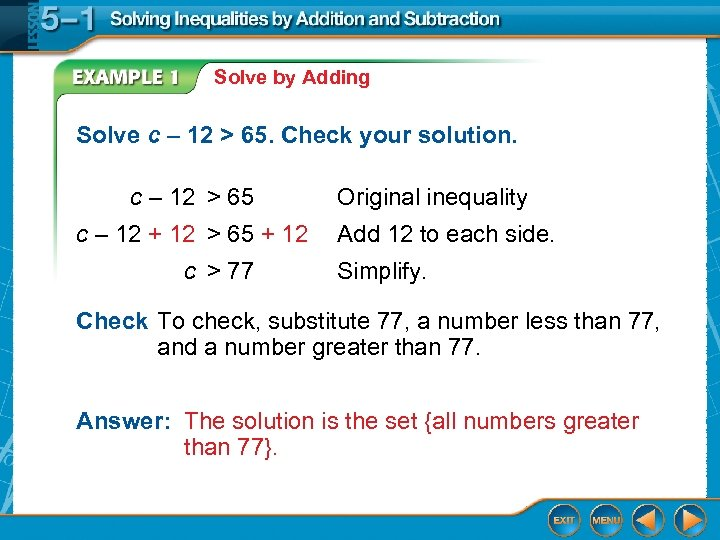Solve by Adding Solve c – 12 > 65. Check your solution. c – 12 > 65 c – 12 + 12 > 65 + 12 c > 77 Original inequality Add 12 to each side. Simplify. Check To check, substitute 77, a number less than 77, and a number greater than 77. Answer: The solution is the set {all numbers greater than 77}.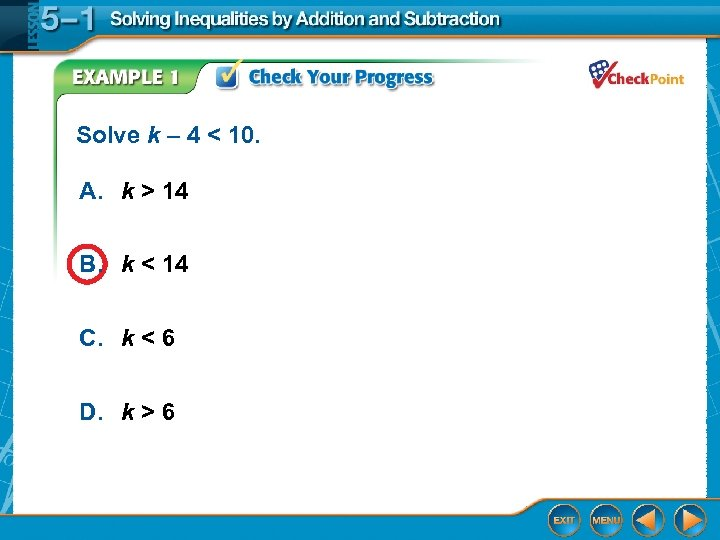Solve k – 4 < 10. A. k > 14 B. k < 14 C. k < 6 D. k > 6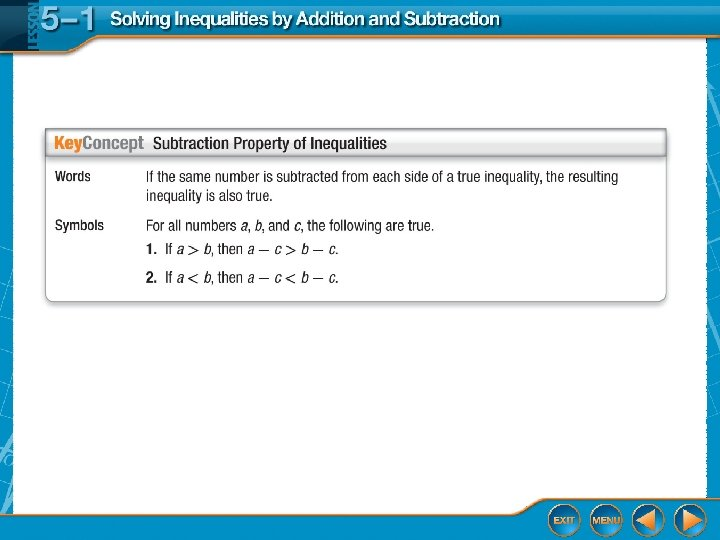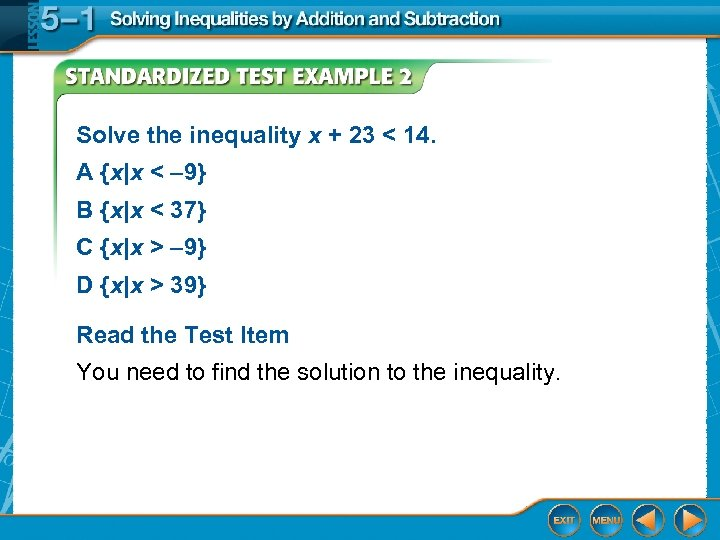Solve the inequality x + 23 < 14. A {x|x < – 9} B {x|x < 37} C {x|x > – 9} D {x|x > 39} Read the Test Item You need to find the solution to the inequality.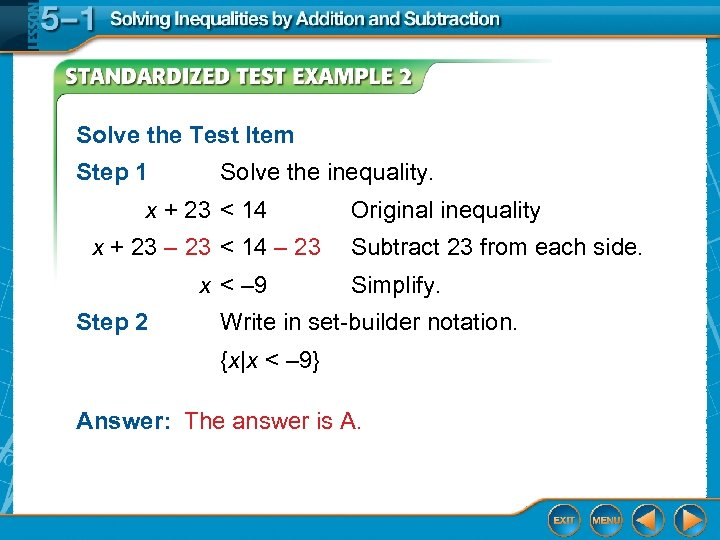Solve the Test Item Step 1 Solve the inequality. x + 23 < 14 x + 23 – 23 < 14 – 23 x < – 9 Step 2 Original inequality Subtract 23 from each side. Simplify. Write in set-builder notation. {x|x < – 9} Answer: The answer is A.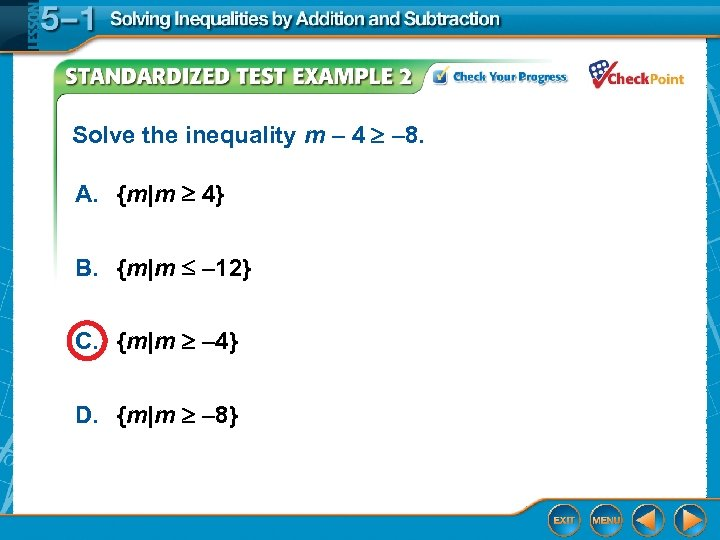Solve the inequality m – 4 – 8. A. {m|m 4} B. {m|m – 12} C. {m|m – 4} D. {m|m – 8}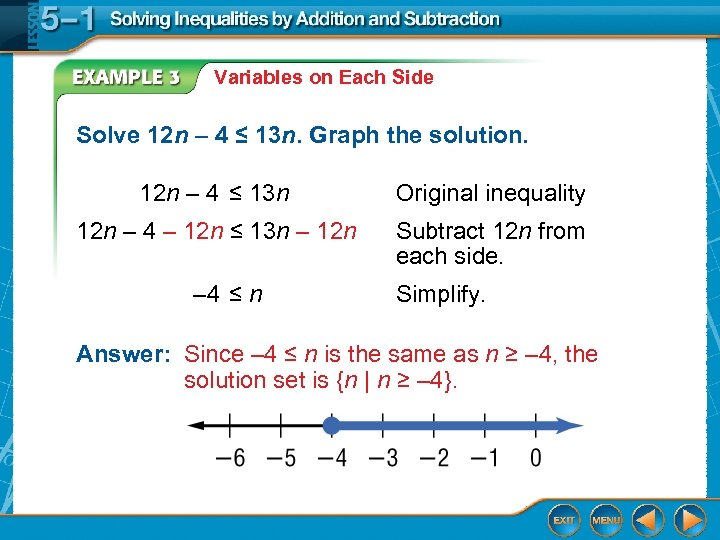Variables on Each Side Solve 12 n – 4 ≤ 13 n. Graph the solution. 12 n – 4 ≤ 13 n Original inequality 12 n – 4 – 12 n ≤ 13 n – 12 n Subtract 12 n from each side. – 4 ≤ n Simplify. Answer: Since – 4 ≤ n is the same as n ≥ – 4, the solution set is {n | n ≥ – 4}.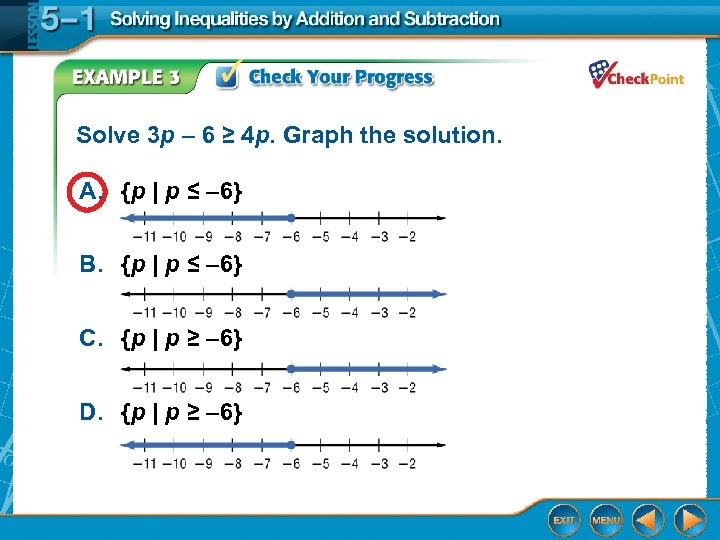Solve 3 p – 6 ≥ 4 p. Graph the solution. A. {p | p ≤ – 6} B. {p | p ≤ – 6} C. {p | p ≥ – 6} D. {p | p ≥ – 6}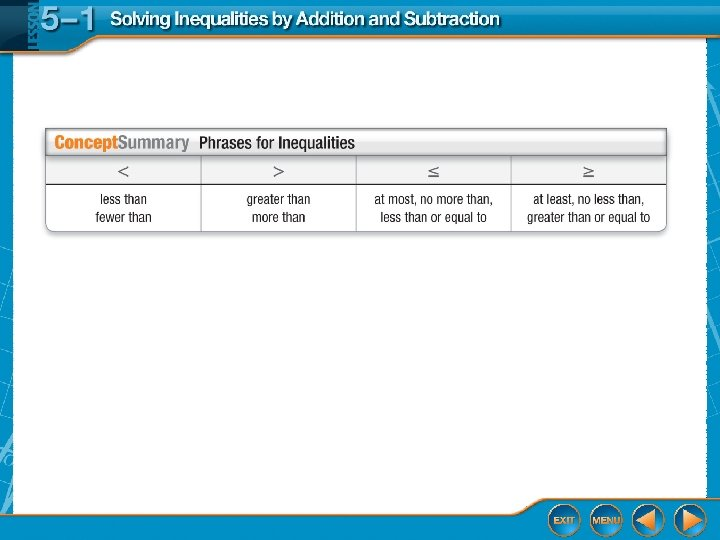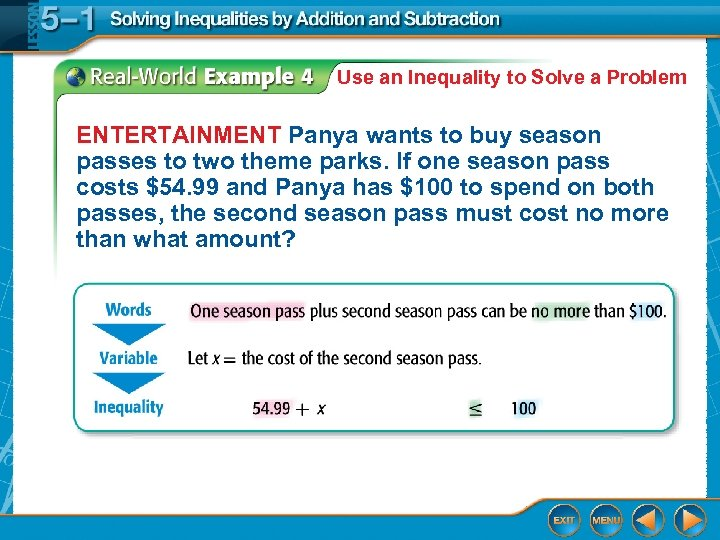Use an Inequality to Solve a Problem ENTERTAINMENT Panya wants to buy season passes to two theme parks. If one season pass costs \$54. 99 and Panya has \$100 to spend on both passes, the second season pass must cost no more than what amount?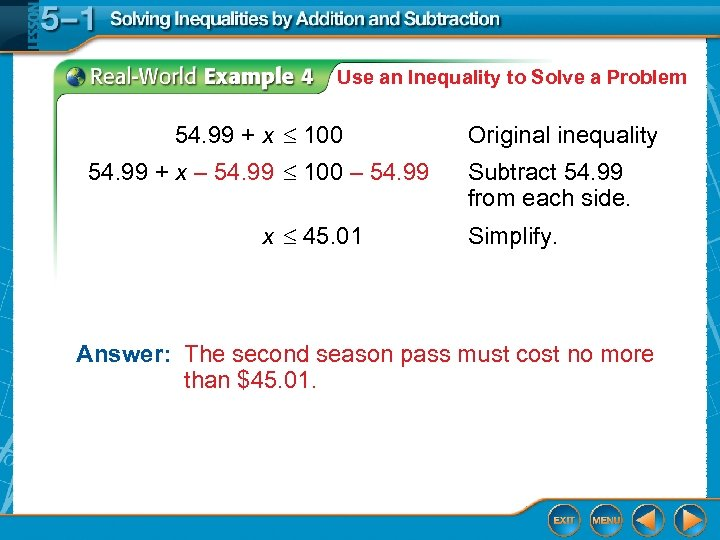Use an Inequality to Solve a Problem 54. 99 + x 100 54. 99 + x – 54. 99 100 – 54. 99 x 45. 01 Original inequality Subtract 54. 99 from each side. Simplify. Answer: The second season pass must cost no more than \$45. 01.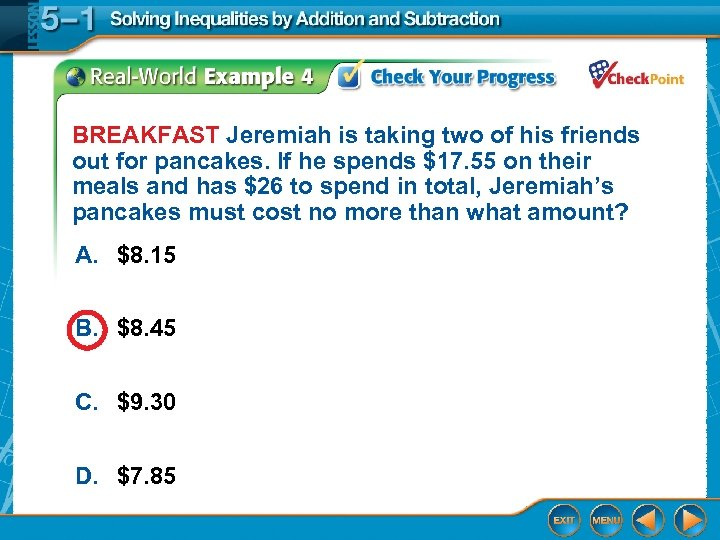BREAKFAST Jeremiah is taking two of his friends out for pancakes. If he spends \$17. 55 on their meals and has \$26 to spend in total, Jeremiah’s pancakes must cost no more than what amount? A. \$8. 15 B. \$8. 45 C. \$9. 30 D. \$7. 85